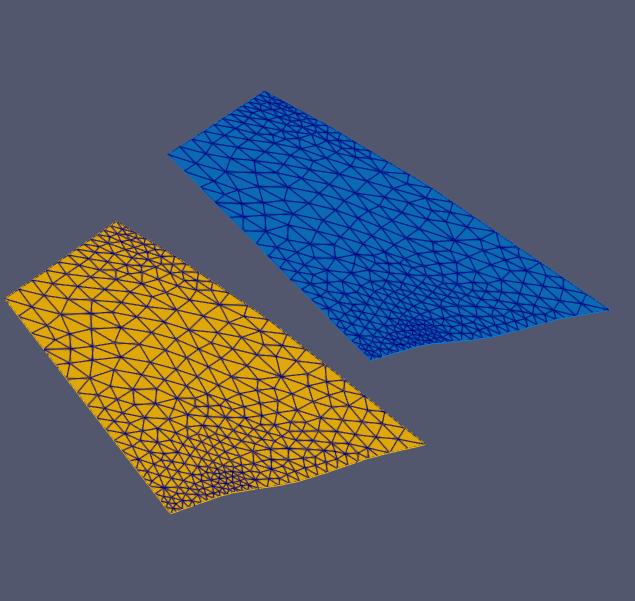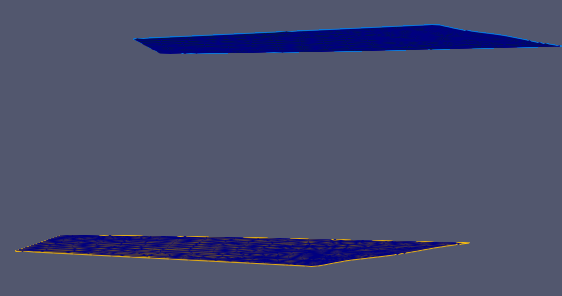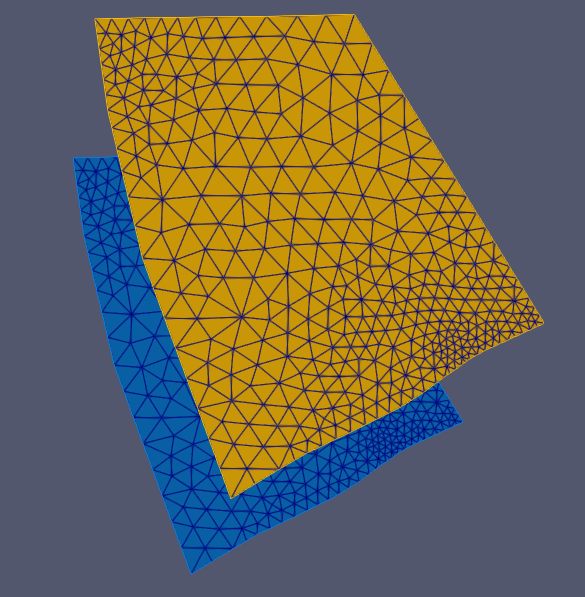# MoveMesh

## Description

MoveMesh is a tool to translate a mesh according to a displacement vector. The displacement vector is defined via input parameters -x, -y, -z.

## Usage

   MoveMesh  [-o <string>] [-z <floating point number>]
[-y <floating point number>]
[-x <floating point number>]
-m <string> [--] [--version] [-h]

Where:

-o <string>,  --output-mesh <string>
output mesh file

-z <floating point number>,  --z <floating point number>
displacement in z direction

-y <floating point number>,  --y <floating point number>
displacement in y direction

-x <floating point number>,  --x <floating point number>
displacement in x direction

-m <string>,  --mesh <string>
(required)  input mesh file

--,  --ignore_rest
Ignores the rest of the labeled arguments following this flag.

--version
Displays version information and exits.

-h,  --help
Displays usage information and exits.

## Example:

Moving the mesh by the displacement vector v = (120,-250,485).

MoveMesh -m mesh.vtu -o mesh_moved.vtu -x 120 -y -250 -z 485Fig.1 Three images showing the same meshes from different point of views (POV). The yellow mesh depicts the input mesh and the blue one the output mesh. The upper image is from an isometric POV, the center image is the view along the x-axis POV and the lower one along the z-axis.

This article was written by Julian Heinze. If you are missing something or you find an error please let us know.
Generated with Hugo 0.117.0 in CI job 387530 | Last revision: November 7, 2023